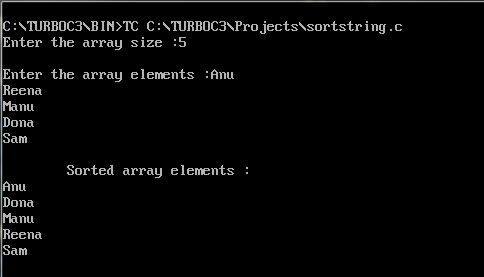UncleCoder.com

UncleCoder.com

Free programming examples and instructions

# C program to sort a given set of strings

## C program for how to sort a given set of strings

by Krishna

Posted on 27 Jul 2018 Category: c Views: 1927

C program to sort a given set of strings

In this program user would be asked to enter a set of Strings and the program would sort and display them in ascending alphabetical order.

``````#include<stdio.h>
#include<string.h>
#include<conio.h>
int main(){
int i,j,count;
char str,temp;
puts("Enter the array size : ");
scanf("%d",&count);

puts("Enter the array elements : ");
for(i=0;i<=count;i++)
gets(str[i]);
for(i=0;i<=count;i++)
for(j=i+1;j<=count;j++){
if(strcmp(str[i],str[j])>0){
strcpy(temp,str[i]);
strcpy(str[i],str[j]);
strcpy(str[j],temp);
}
}
printf("\t\tSorted array elements :");
for(i=0;i<=count;i++)
puts(str[i]);

return 0;
getch();
}
``````

OUTPUT### Latest posts in c

##### C program to find largest among two numbers• View all

• View all

• View all

• View all

• View all